Question

# A standard pair of six-sided dice is rolled. What is the probability of rolling a sum...

A standard pair of six-sided dice is rolled. What is the probability of rolling a sum greater than 9? Express your answer as a fraction or a decimal number rounded to four decimal places.

The sample space is,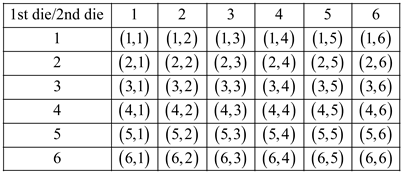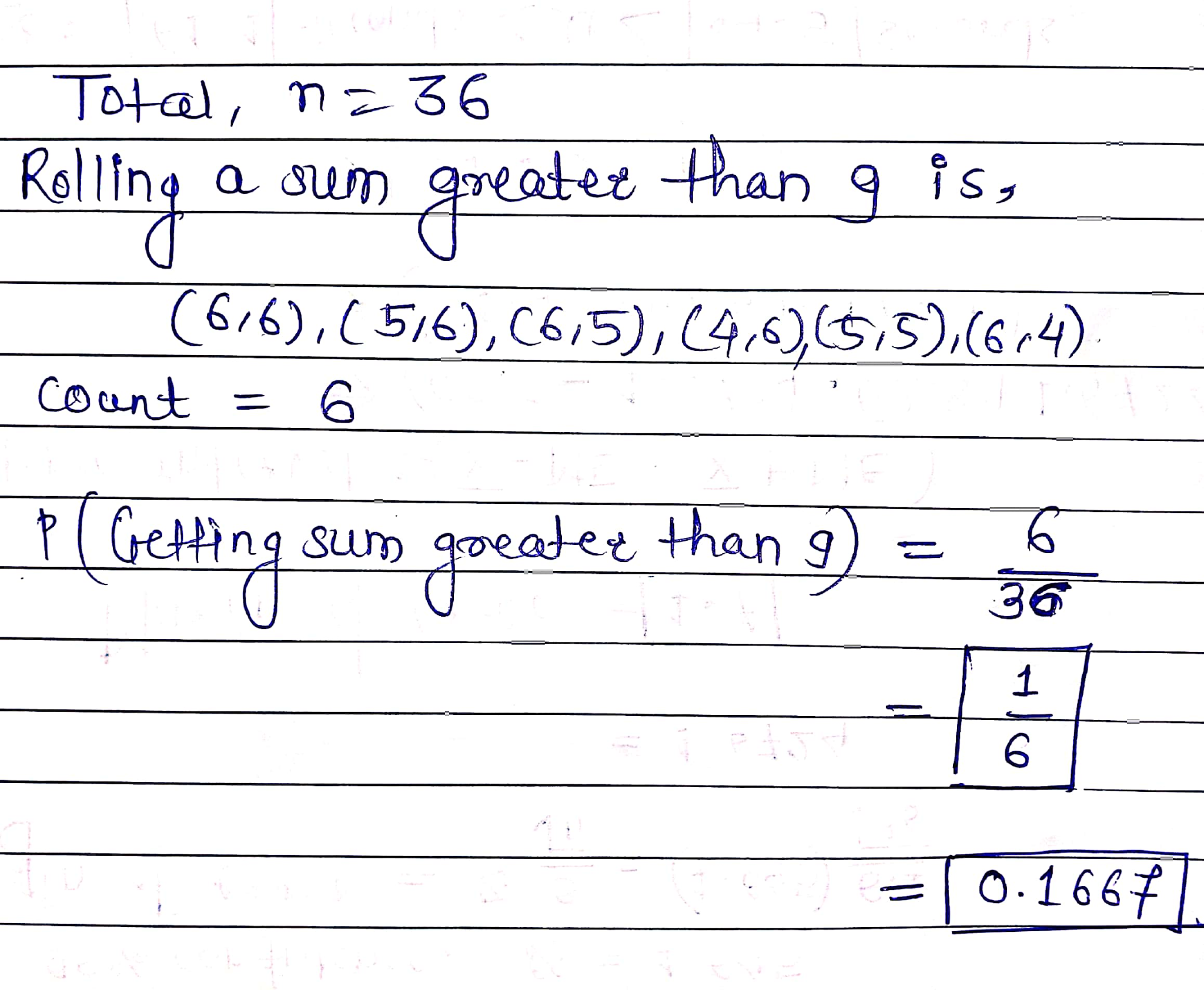5/18 or 0.2778
source: Hawks learning

#### Earn Coins

Coins can be redeemed for fabulous gifts.

Similar Homework Help Questions
• ### A standard pair of six-sided dice is rolled. What is the probability of rolling a sum...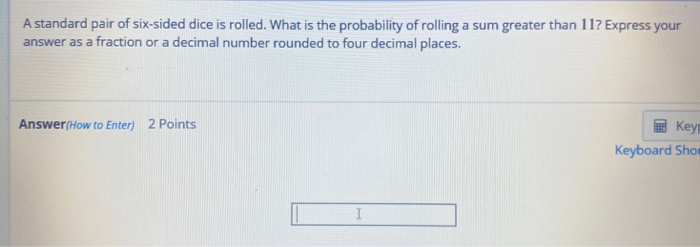A standard pair of six-sided dice is rolled. What is the probability of rolling a sum greater than 117 Express your answer as a fraction or a decimal number rounded to four decimal places. Answer/How to Enter) 2 Points Key Keyboard Shor I

• ### A standard pair of six-sided dice is rolled. What is the probability of rolling a sum...

A standard pair of six-sided dice is rolled. What is the probability of rolling a sum less than 5? Express your answer as a fraction or a decimal number rounded to four decimal places.

• ### Correct A standard pair of six-sided dice is rolled. What is the probability of rolling a...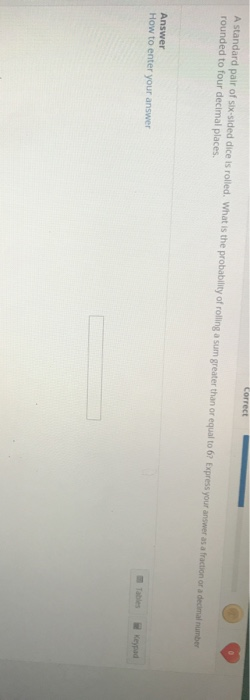Correct A standard pair of six-sided dice is rolled. What is the probability of rolling a sum greater than or equal to 62 Express your answer as a fraction or a decimal number rounded to four decimal places Answer How to enter your answer

• ### What is the probability of rolling a sum of 6 on a standard pair of six-sided...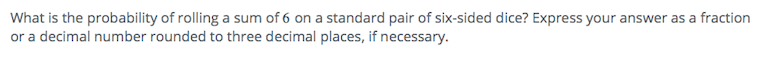What is the probability of rolling a sum of 6 on a standard pair of six-sided dice? Express your answer as a fraction or a decimal number rounded to three decimal places, if necessary.

• ### 1. A standard six-sided die is rolled. What is the probability of rolling a number greater...

1. A standard six-sided die is rolled. What is the probability of rolling a number greater than 3? Express your answer as a simplified fraction or a decimal rounded to four decimal places. Answer: ____________________ 2. A box contains 48 red marbles, 53 white marbles, and 67 blue marbles. If a marble is randomly selected from the box, what is the probability that it is blue? Express your answer as a simplified fraction or a decimal rounded to four decimal...

• ### When a standard pair of six sided dice are rolled, what is the probability of getting...When a standard pair of six sided dice are rolled, what is the probability of getting a 6? Express your answer as a decimal rounded to the thousandsth place.)

• ### A pair of dice is rolled. Find the probability of rolling a) The probability of rolling...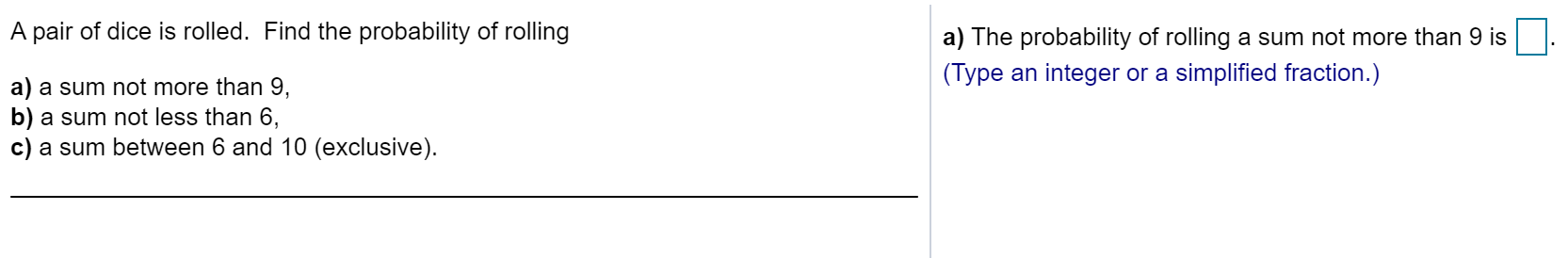A pair of dice is rolled. Find the probability of rolling a) The probability of rolling a sum not more than 9 is (Type an integer or a simplified fraction.) a) a sum not more than 9, b) a sum not less than 6, c) a sum between 6 and 10 (exclusive).

• ### If two six sided dice are rolled, what is the probability of rolling a 5 on...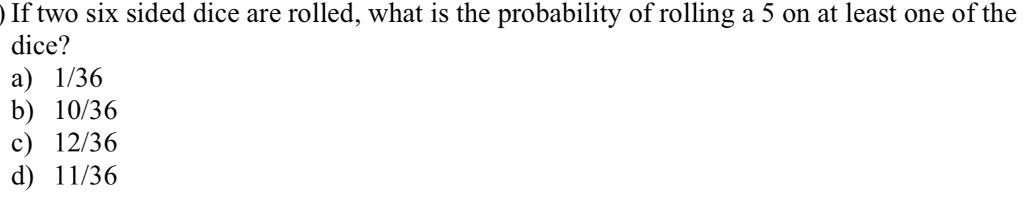If two six sided dice are rolled, what is the probability of rolling a 5 on at least one of the dice? a) I/36 b) 10/36 c) 12/36 d) 11/36

• ### A single six sided dice is rolled. Find the probability of rolling a seven

A single six sided dice is rolled. Find the probability of rolling a seven

• ### What is the marginal probability of rolling a 2 using two six-sided dice? Round your answer...

What is the marginal probability of rolling a 2 using two six-sided dice? Round your answer to three decimal places What is the probability of rolling something other than double 2s using two six-sided dice? Round your answer to three decimal places